# Chapter 2: Models in one dimension

 Page 4/25 Date 09.06.2018 Size 2.45 Mb.

## Section 2.1: Heat flow in a bar; Fourier's Law

MATLAB can compute both indefinite and definite integrals. The command for computing an indefinite integral (antiderivative) is exactly analogous to that for computing a derivative:

syms x

f=x^2

f =

x^2

int(f,x)

ans =

x^3/3
As this example shows, MATLAB does not add the "constant of integration." It simply returns one antiderivative (when possible). If the integrand is too complicated, MATLAB just returns the integral unevaluated, and prints a warning message.
int(exp(cos(x)),x)

Warning: Explicit integral could not be found.

ans =

int(exp(cos(x)), x)

To compute a definite integral, we must specify the limits of integration:
int(x^2,x,0,1)

ans =

1/3
MATLAB has commands for estimating the value of a definite integral that cannot be computed analytically. Consider the following example:
int(exp(cos(x)),x,0,1)

Warning: Explicit integral could not be found.

ans =

int(exp(cos(x)), x = 0..1)

Since MATLAB could not find an explicit antiderivative, I can use the quad function to estimate the definite integral. The quad command takes, as input, a function rather than an expression (as does int). Therefore, I must first create a function:
f=@(x)exp(cos(x))

f =

@(x)exp(cos(x))

ans =

2.3416
As a further example of symbolic calculus, I will use the commands for integration and differentiation to test Theorem 2.1 from the text. The theorem states that (under certain conditions) a partial derivative can be moved under an integral sign: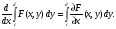Here is a specific instance of the theorem:
syms x y c d

f=x*y^3+x^2*y

f =

x^2*y + x*y^3

r1=diff(int(f,y,c,d),x)

r1 =

- (x*(c^2 - d^2))/2 - ((c^2 - d^2)*(c^2 + d^2 + 2*x))/4
r2=int(diff(f,x),y,c,d)

r2 =

-((c^2 - d^2)*(c^2 + d^2 + 4*x))/4
r1-r2

ans =

((c^2 - d^2)*(c^2 + d^2 + 4*x))/4 - ((c^2 - d^2)*(c^2 + d^2 + 2*x))/4 - (x*(c^2 - d^2))/2

simplify(ans)

ans =

0

### Solving simple boundary value problems by integration

Consider the following BVP: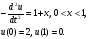The solution can be found by two integrations (cf. Example 2.2 in the text). Remember, MATLAB does not add a constant of integration, so I do it explicitly:
clear

syms x C1 C2

int(-(1+x),x)+C1

ans =

C1 - (x + 1)^2/2
int(ans,x)+C2

ans =

C2 + C1*x - (x + 1)^3/6
u=ans

u =

C2 + C1*x - (x + 1)^3/6
The above function u, with the proper choice of C1 and C2, is the desired solution. To find the constants, I solve the (algebraic) equations implied by the boundary conditions. The MATLAB command solve can be used for this purpose.

### The MATLAB solve command

Before completing the previous example, I will explain the solve command on some simpler examples.

Suppose I wish to solve the linear equation ax+b=0 for x. I can regard this as a root-finding problem: I want the root of the function f(x)=ax+b. The solve command finds the root of a function with respect to a given independent variable:
syms f x a b

f=a*x+b

f =

b + a*x

solve(f,x)

ans =

-b/a
If the equation has more than one solution, solve returns the possibilities in an array:
syms f x

f=x^2-3*x+2;

solve(f,x)

ans =

1

2

As these examples show, solve is used to find solutions of equations of the form f(x)=0; only the expression f(x) is input to solve.

solve can also be used to solve a system of equations in several variables. In this case, the equations are listed first, followed by the unknowns. For example, suppose I wish to solve the equations
x+y=1,

2x-y=1.
Here is the command:

syms x y

s=solve(x+y-1,2*x-y-1,x,y)

s =

x: [1x1 sym]

y: [1x1 sym]
What kind of variable is the output s? If we list the variables in the workspace,
whos

Name Size Bytes Class Attributes

C1 1x1 60 sym

C2 1x1 60 sym

a 1x1 60 sym

ans 2x1 60 sym

b 1x1 60 sym

f 1x1 60 sym

s 1x1 368 struct

u 1x1 60 sym

x 1x1 60 sym

y 1x1 60 sym

we see that s is a 1 by 1 struct array, that is, an array containing a single struct. A struct is a data type with named fields that can be accessed using the syntax variable.field. The variable s has two fields:
s

s =

x: [1x1 sym]

y: [1x1 sym]

The two fields hold the values of the unknowns x and y:
s.x

ans =

2/3

s.y

ans =

1/3
If the system has more than one solution, then the output of solve will be a struct, each of whose fields is an array containing the values of the unknowns. Here is an example:

s=solve(x^2+y^2-1,y-x^2,x,y)

s =

x: [4x1 sym]

y: [4x1 sym]

The first solution is
pretty(s.x(1))

/ 1/2 \1/2

| 5 |

| ---- - 1/2 |

\ 2 /
pretty(s.y(1))

1/2

5

---- - 1/2

2
The second solution is
pretty(s.x(2))

/ 1/2 \1/2

| 5 |

| - ---- - 1/2 |

\ 2 /

pretty(s.y(2))

1/2

5

- ---- - 1/2

2
You might notice that the second solution is complex (at least, the value of x is complex). This might be easier to see from the numerical value of the expressions, which we can see with the double command (which converts a symbolic expression to a floating point value, if possible)
double(s.x(2))

ans =

0 + 1.2720i

double(s.y(2))

ans =

-1.6180
Here are the remaining solutions (given in floating point)

double(s.x(3))

ans =

-0.7862

double(s.y(3))

ans =

0.6180
double(s.x(4))

ans =

0 - 1.2720i

double(s.y(4))

ans =

-1.6180
If the equation to be solved cannot be solved exactly, then solve automatically tries to solve it numerically, using extended precision arithmetic (approximately 32 decimal digits):
syms x

solve(x^5+sym(4)*x^4-x^3+x^2-x+1,x)

ans =

-4.3019656878883790704888463433324

0.40236742000277868343510597733001*sqrt(-1) + 0.49445817238673908475778249075192

- 0.67380934595293810995276180453239*sqrt(-1) - 0.34347532844254954951335931908572

0.67380934595293810995276180453239*sqrt(-1) - 0.34347532844254954951335931908572

0.49445817238673908475778249075192 - 0.40236742000277868343510597733001*sqrt(-1)

Finally, there is another way to call solve. The equation and unknowns can be given as strings, meaning that there is no need to define symbolic variables before calling solve:
solve('x^2-2*x-1=0','x')

ans =

2^(1/2) + 1

1 - 2^(1/2)

Notice that, when specifying the equation in symbolically form, it must be expressed with the right-hand side equal to 0, and only the left-hand side is passed to solve (that is, to solve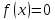for , we call solve as solve(f(x),x). However, when calling solve using strings instead of symbolic expressions, we can use either form,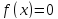(as above) or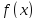:
solve('x^2-2*x-1','x')

ans =

2^(1/2) + 1

1 - 2^(1/2)

#### Back to the example

We can now solve for the constants of integrations in the solution to the BVPRecall that the solution is of the form
u

u =

C2 + C1*x - (x + 1)^3/6
We must use the boundary conditions to compute C1 and C2. The equations are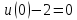and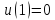. Notice how I use the subs command to form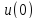and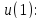s=solve(subs(u,x,0)-2,subs(u,x,1),C1,C2)

s =

C1: [1x1 sym]

C2: [1x1 sym]

Here are the values of C1 and C2:
s.C1

ans =

-5/6
s.C2

ans =

13/6
Here is the solution:
u=subs(u,{C1,C2},{s.C1,s.C2})

u =

13/6 - (x + 1)^3/6 - (5*x)/6
Notice how, when substituting for two or more variables, the variables and the values are enclosed in curly braces.
Let us check our solution:
-diff(u,x,2)

ans =

x + 1

subs(u,x,sym(0))

ans =

2

subs(u,x,sym(1))

ans =

0
The differential equation and the boundary conditions are satisfied.

#### Another example

Now consider the BVP with a nonconstant coefficient: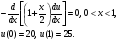Integrating once yields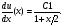(It is easier to perform this calculation in my head than to ask MATLAB to integrate 0.) I now perform the second integration:
clear

syms C1 C2 x

u=int(C1/(1+x/2),x)+C2

u =

C2 + 2*C1*log(x + 2)
Now I use solve to find C1 and C2:
s=solve(subs(u,x,0)-20,subs(u,x,1)-25,C1,C2)

s =

C1: [1x1 sym]

C2: [1x1 sym]

Here is the solution:
u=subs(u,{C1,C2},{s.C1,s.C2})

u =

(45035996273704960*log(x + 2))/3652105019575333 + 41825526550679865/3652105019575333
Notice the unexpected answer; the problem is that MATLAB did not interpret the constants in the equations (0, 1, 20, 25) as symbolic quantities, but rather as numerical (floating point) values. As a result, it used extended precision arithmetic while finding a solution. The desired solution can be found by specifying that the various numbers be treated as symbolic:
clear u

syms C1 C2 x

u=int(C1/(1+x/2),x)+C2

u =

C2 + 2*C1*log(x + 2)
s=solve(subs(u,x,sym(0))-sym(20),subs(u,x,sym(1))-sym(25),C1,C2)

s =

C1: [1x1 sym]

C2: [1x1 sym]

u=subs(u,{C1,C2},{s.C1,s.C2})

u =

(5*(5*log(2) - 4*log(3)))/(log(2) - log(3)) - (5*log(x + 2))/(log(2) - log(3))
Now I will check the answer:
simplify(-diff((1+x/2)*diff(u,x),x))

ans =

0
subs(u,x,0)

ans =

20

subs(u,x,1)

ans =

25.0000